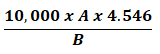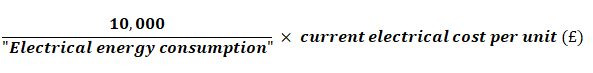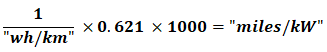# New Car Fuel Consumption & Emission Figures

Contents

## Fuel cost

The fuel cost of driving 10,000 miles is calculated using the combined fuel consumption figure and the respective average fuel figures. The fuel figures used are those published by the Department for Business, Energy and Industrial Strategy and appear on the ‘efficiency labels’ that can be seen in car showrooms. There are of course many changes to fuel prices during the course of a year, not to mention many regional variations. It is because of this that we have decided to use the average figure, taken at a given point in the year, as this ensures a level playing field for comparison purposes.

### The fuel costs are calculated as follows:A = The current cost per litre of Petrol, Diesel or LPG (as applies to the car), e.g. 147p, 167p or 82p

B = The Imperial combined Fuel Consumption figure (MPG)

4.546 = The figure for conversion of litres to imperial gallons.

### The Electric costs are calculated as follows:### The “Electrical energy consumption”  is calculated as follows:wh/km is taken from the vehicles Certificate of Conformity (CoC)

### Total cost 10000 miles

This is a combination of the fuel cost + the electricity cost